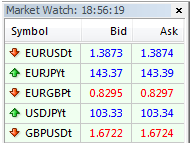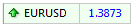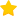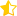# What is a pip?

The word “pip”, initially going back to an acronym PIP which means Point In Percentage, is now an absolutely normal word, one of the most frequently used by traders and investors in Forex industry.

A pip is the smallest unit of currency rate change. While trading in the Forex market, you will have to closely monitor the currency rates and how they change. You will be able to do so easily using the Market Watch panel of your MetaTrader 4 terminal:You have probably noticed that the panel shows the currency exchange rate, bid and ask, which is constantly changing, and this rate is usually a fraction consisting of an integer number and some numbers that follow after the point. Now, one pip represents one ten-thousandth of the currency rate, though for JPY and some other rates it is one-hundredth. Thus, the amount of pips reflects how much the price changed and, eventually, how much you are profiting or losing.

Let’s look on the screenshot below:Here you can see the EUR/USD currency rate, which right now is 1.3873. If it moves one pip up, it’ll be 1.3874, if one pip down, 1.3872. So, this is, as said above, the minimum change reflected in the currency pair, one ten-thousandth of the rate or the fourth digit after the point (for JPY rates, it will be the second digit).

## How to Calculate Pip Value?

Now that we know what a pip is, you may ask: so what’s next? Why should I need those pips?

The answer is that you should need them to know your profit and loss. In the Forex market, profits and losses are generally measured by pips, so you would often hear traders say “I made a 150-pip profit” or “This was a 240-pip loss”. To convert the amount of pips into the amount of cash and to know exactly how much money you won or lost, you must first learn how to calculate the pip value.

### The general formula for that is:

Position size/10,000 (for any rate except that involving JPY)

Position size/100 (for a JPY rate)

Now, there are three possible position sizes:

1)      Standard lot (when you trade 100,000 units of base currency)

2)      Mini lot (10,000 units of base currency)

3)      Micro lot (1,000 units of base currency)

Thus, if we buy a EUR/USD standard lot, we get 100,000/10,000=10. The result is always in counter currency (the second one in the pair), so here we get that 1 pip of EUR/USD costs 10 American dollars.

Let’s take another example. Suppose we bought a EUR/GBP micro lot; what will be the pip value?

Applying our easy formula, we now get 1,000/10,000=0.1, i.e. 0.10 British pounds (10 pence). Now you know that, say, if you make a 300-pip profit while trading this pair, it will be 300*0.10=30 pounds.

Rate this post:(18 votes, average: 3.83 out of 5)Loading...
---